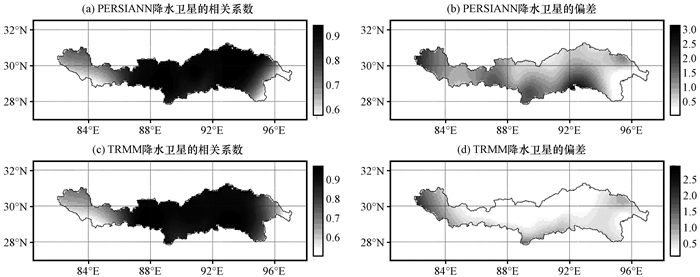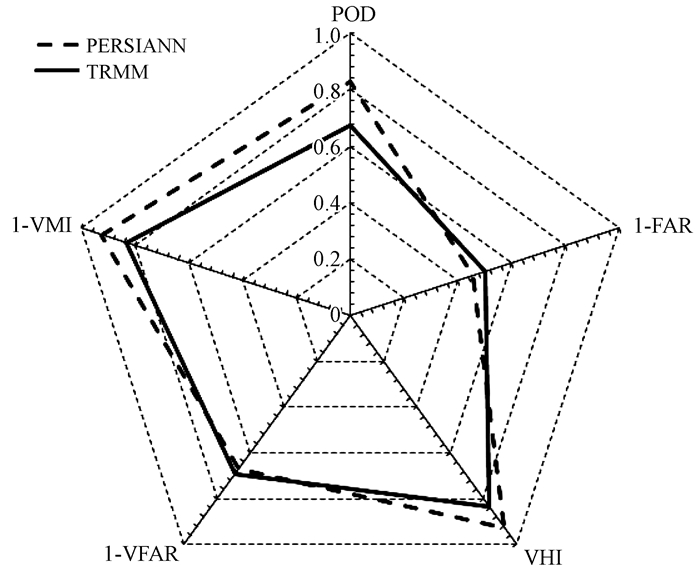﻿ 不同降水卫星数据反演降水量精度评价——以雅鲁藏布江流域为例
 高原气象2019, Vol. 38Issue (2): 386-396  DOI: 10.7522/j.issn.1000-0534.2018.000920

### 引用本文 [复制中英文]

[复制中文]
Liu Jiangtao, Xu Zongxue, Zhao Huan, et al. 2019. Accuracy Assessment for Two Satellite Precipitation Products: Case Studies in the Yarlung Zangbo River Basin[J]. Plateau Meteorology, 38(2): 386-396. DOI: 10.7522/j.issn.1000-0534.2018.00092.
[复制英文]

### 文章历史

1. 北京师范大学水科院研究院, 北京 100875;
2. 城市水循环与海绵城市技术北京市重点实验室, 北京 100875

1 引言

2 研究区概况与资料选取 2.1 研究区概况图 1 雅鲁藏布江流域位置及气象站点分布 Fig. 1 The distribution of meteorological stations in the Yarlung Zangbo River basin
2.2 资料选取表 1 气象卫星的时空分辨率和可用数据时间段 Table 1 Characteristics of the two satellite products
3 研究方法

3.1 降水评估定量指标

(1) 偏差(Bias)

 $Bias=\frac{\sum\limits_{i=1}^{n}\left(S I M_{i}-O B S_{i}\right)}{\sum\limits_{i=1}^{n} O B S_{i}},$ (1)

(2) 相关系数(Corr)

 $Corr=\frac{\sum\limits_{i=1}^{n}\left(S M_{i}-\overline{S I M}\right)\left(O B S_{i}-\overline{O B S}\right)}{\sqrt{\sum\limits_{i=1}^{n}\left(S M_{i}-\overline{S M}\right)^{2} \cdot \sum\limits_{i=1}^{n}\left(O B S_{i}-\overline{O B S}\right)^{2}}},$ (2)

3.2 降水评估分类指标表 2 地面降水事件和卫星捕捉降水事件发生情况表 Table 2 The contingency table for satellite products

(1) 探照率(POD)

POD(Probability of Detection)是指降水卫星能正确的探测到的降水事件的次数在所有降水事件次数中的比例。

 $P O D=\frac{\text { hit }\_ \text { num }}{\text { hit }_{\text { - }} \text { num }+\text { miss }\_ \text { num }},$ (3)

(2) 错报率(FAR)

FAR(False Alarm Ration)是指卫星误将无降水事件识别为降水事件的次数在卫星观测到降水事件总数中的比例, 表示卫星对降水事件报错的概率。

 $F A R=\frac{\text { false }\_ \text { num }}{\text { false }\_ \text { num }+\text { hit }\_ \text { num }},$ (4)

3.3 降水量体积分类指标

(1) 降水量探照率(Volumetric Hit Index, VHI)

 $\mathit{VHI}{\rm{ = }}\frac{{\sum\limits_{i = 1}^n {\left[ {{\rm{SI}}{{\rm{M}}_i}|\left({{\rm{SI}}{{\rm{M}}_i} > {\rm{thr\& OB}}{{\rm{S}}_i} > {\rm{thr}}} \right)} \right]} }}{{\sum\limits_{i = 1}^n {\left[ {{\rm{SI}}{{\rm{M}}_i}|\left({{\rm{SI}}{{\rm{M}}_i} > {\rm{thr\& OB}}{{\rm{S}}_i} > {\rm{thr}}} \right)} \right]} + \sum\limits_{i = 1}^n {\left[ {{\rm{OB}}{{\rm{S}}_i}|\left({{\rm{SI}}{{\rm{M}}_i} \le {\rm{thr\& OB}}{{\rm{S}}_i} > {\rm{thr}}} \right)} \right]} }},$ (5)

(2) 降水量错报率(Volumetric False Alarm Ratio, VFAR)

 $\mathit{VFAR}{\rm{ = }}\frac{{\sum\limits_{i = 1}^n {\left[ {{\rm{SI}}{{\rm{M}}_i}|\left({{\rm{SI}}{{\rm{M}}_i} > {\rm{thr\& OB}}{{\rm{S}}_i} \le {\rm{thr}}} \right)} \right]} }}{{\sum\limits_{i = 1}^n {\left[ {{\rm{SI}}{{\rm{M}}_i}|\left({{\rm{SI}}{{\rm{M}}_i} > {\rm{thr\& OB}}{{\rm{S}}_i} > {\rm{thr}}} \right)} \right]} + \sum\limits_{i = 1}^n {\left[ {{\rm{OB}}{{\rm{S}}_i}|\left({{\rm{SI}}{{\rm{M}}_i} > {\rm{thr\& OB}}{{\rm{S}}_i} \le {\rm{thr}}} \right)} \right]} }},$ (6)

VFAR是指标FAR的拓展, 表示在阈值thr以上的降水量被卫星误判的比例, 它的数值范围为0~1, 其值愈接近于0, 表示降水量被卫星误判的可能愈小。

(3) 降水量漏报率(Volumetric Miss Index, VMI)

 $\mathit{VMI}{\rm{ = }}\frac{{\sum\limits_{i = 1}^n {\left[ {{\rm{OB}}{{\rm{S}}_i}|\left({{\rm{SI}}{{\rm{M}}_i} > {\rm{thr\& OB}}{{\rm{S}}_i} > {\rm{thr}}} \right)} \right]} }}{{\sum\limits_{i = 1}^n {\left[ {{\rm{SI}}{{\rm{M}}_i}|\left({{\rm{SI}}{{\rm{M}}_i} > {\rm{thr\& OB}}{{\rm{S}}_i} > {\rm{thr}}} \right)} \right]} + \sum\limits_{i = 1}^n {\left[ {{\rm{OB}}{{\rm{S}}_i}|\left({{\rm{SI}}{{\rm{M}}_i} \le {\rm{thr\& OB}}{{\rm{S}}_i} > {\rm{thr}}} \right)} \right]} }},$ (7)

VMI表示在阈值thr以上的降水量被卫星漏报的比例, 它的数值范围为0~1, 其值愈接近于0, 表示降水量被卫星漏报的可能愈小。

4 结果与分析 4.1 不同降水区间内卫星数据与站点数据偏差分析图 2 不同降水量范围内的降水卫星数据和地面站点的数据之间的平均偏差分布 Fig. 2 Distribution of mean bias between satellite data and stations data for different precipitation ranges

4.2 卫星降水数据与站点降水数据定量分析 4.2.1 卫星降水数据精度评估图 3 1998—2015年PERSIANN卫星和TRMM卫星降水数据与地面站点数据密度散点分布 P＜0.05表示通过95%信度水平检验 Fig. 3 Precipitation scatter density diagram between satellite data and stations data in the Yarlung Zangbo River basin during 1998—2015
4.2.2 卫星降水数据站点精度检验表 3 雅鲁藏布江流域1998—2015年PERSIANN、TRMM数据与地面站点的数据的相关系数和偏差 Table 3 Coefficient of correlation and deviation of the two satellite products in the Yarlung Zangbo River basin图 4 雅鲁藏布江流域1998—2015年卫星数据与地面站点数据的相关系数(左)和偏差(右)空间分布 Fig. 4 Distribution of correlation coefficient (left) and bias (right) between satellite data and stations data in the Yarlung Zangbo River basin during 1998—2015

4.3 卫星降水数据与站点降水数据分类指标比较分析

4.3.1 卫星降水数据整体精度检验图 5 PERSIANN和TRMM降水卫星分类指标 Fig. 5 Indices in detecting precipitation between PERSIANN and TRMM

POD表示降水卫星能正确探测到的降水事件的次数在所有降水事件次数中的比例, PERSIANN卫星的POD值为0.830, TRMM卫星的POD值为0.674。PERSIANN卫星比TRMM卫星的POD高了23.1%, 说明PERSIANN降水卫星能够捕捉到雅鲁藏布江流域发生的绝大部分降水事件, 而TRMM降水卫星遗漏了相当一部分降水事件。

VHI数值大小反映流域的降水事件中有多少降水量可以被卫星正确探测到的比例, PERSIANN降水卫星的VHI为0.927, TRMM卫星的VHI为0.833, 说明PERSIANN降水卫星捕捉降水量的能力同样比TRMM卫星捕捉降水量的能力强。

FAR反映了卫星将无降水事件识别为降水事件的次数在卫星观测到降水事件总数中的比例。PERSIANN和TRMM卫星的FAR值分别为0.54和0.50。VFAR数值大小反映流域的降水事件中有多少降水量可以被卫星错误判断的比例, PERSIANN降水卫星的VFAR值为0.33, 而TRMM降水卫星的VFAR为0.31, 两个卫星对降水量的“误判”情况类似。PERSIANN降水卫星在减少误报降水事件的能力上略差于TRMM, 但是差别不大。这可能与TRMM卫星搭载了TMI(微波成像仪)、测雨雷达PR等传感器有关(Kummerow et al, 1998)。VMI数值大小反映流域的降水事件中有多少降水量可以被卫星漏掉的比例, PERSIANN和TRMM卫星的VMI值分别为0.073和0.167。PERSIANN卫星能够捕捉更多的降水量, 被其“漏掉”的降水量更少。

4.3.2 卫星降水数据站点精度检验图 6 降水卫星分类指标数值在雅鲁藏布江流域空间分布 Fig. 6 Distribution of indices in detecting precipitation in the Yarlung Zangbo River basin

5 结论

(1) PERSIANN卫星数据和TRMM卫星数据精度存在降水类型不稳定性, 并随着降水量范围的增大而增大。日降水量范围在0~0.5 mm的降水类型中, 两个卫星数据存在明显的高估, PERSIANN卫星数据的偏差在正偏差中占了15%, TRMM卫星数据在正偏差中占了22%。日降水量在9~50 mm的降水范围内, PERSIANN卫星数据的偏差占了总偏差大小的54%, TRMM卫星数据的偏差占了总偏差大小的44.7%。两种降水卫星均在不同程度上对降水的估计有偏差, 总是高估了小降水量, 低估了大降水量, 并且PERSIANN降水卫星数据要比TRMM降水卫星对降水数据偏离程度小。

(2) PERSIANN降水卫星数据的相关系数平均值为0.663, Bias的平均值为0.845。TRMM降水卫星数据的相关系数平均值为0.666, Bias的平均值为0.579。仅从定量指标上看, 两个卫星数据的相关系数差别较小, 精度接近。

(3) 基于分类指标和降水量分类指标的评价指标体系, 可以更加有效的区分PERSIANN卫星和TRMM卫星对降水的反演能力。整体上, PERSIANN卫星的POD值为0.830, VHI为0.927, FAR值为0.54, VFAR值为0.33, VMI值为0.073。TRMM卫星的POD值为0.674, VHI为0.833, FAR值为0.50, VFAR值为0.31, VMI值为0.167。在空间分布上, PERSIANN卫星在各站点的各分类指标优于TRMM卫星的指标。考虑降水量分类指标的评价指标体系比单纯使用传统定量指标评价降水卫星的精度能更加有效地反映出降水卫星对地面降水特征的捕捉能力大小, PERSIANN卫星对降水事件和降水量的反演精度要高于TRMM卫星。

 Aghakouchak A, Mehran A. 2013. Extended contingency table:Performance metrics for satellite observations and climate model simulations[J]. Water Resources Research, 49(10): 7144–7149. DOI:10.1002/wrcr.20498,2013 Alijanian M, Rakhshandehroo G R, Mishra A K, et al. 2017. Evaluation of satellite rainfall climatology using CMORPH, PERSIANN-CDR, PERSIANN, TRMM, MSWEP over Iran[J]. International Journal of Climatology, 37(14): 4896–4914. DOI:10.1002/joc.5131 Dinku T, Ceccato P, Lemma M, et al. 2007. Validation of satellite rainfall products over East Africa's complex topography[J]. International Journal of Remote Sensing, 28(7): 1503–1526. DOI:10.1080/01431160600954688 Hong Y. 2004. Precipitation estimation from remotely sensed information using Artificial Neural Network-Cloud Classification System[J]. Journal of Applied Meteorology, 36(9): 1176–1190. DOI:10.1175/1520-0450(1997)036 Hong Y, Hsu K L, Moradkhani H, et al. 2006. Uncertainty quantification of satellite precipitation estimation and Monte Carlo assessment of the error propagation into hydrologic response[J]. Water Resources Research, 42(8): 2643–2645. DOI:10.1029/2005WR004398,2006 Hu Q, Yang D, Li Z, et al. 2014. Multi-scale evaluation of six high-resolution satellite monthly rainfall estimates over a humid region in China with dense rain gauges[J]. International Journal of Remote Sensing, 35(4): 1272–1294. DOI:10.1175/JAM2173.1 Kummerow C, Barnes W, Kozu T, et al. 1998. The Tropical Rainfall Measuring Mission (TRMM) sensor package[J]. Journal of atmospheric and oceanic technology, 15(3): 809–817. DOI:10.1175/1520-0426(1998)015 Levizzani V, Laviola S, Cattani E. 2011. Detection and measurement of snowfall from space[J]. Remote Sensing, 3(1): 145–166. DOI:10.3390/rs3010145 Smith T M, Arkin P A, Bates J J, et al. 2006. Estimating bias of satellite-based precipitation estimates[J]. Journal of Hydrometeorology, 7(5): 841–856. DOI:10.1175/JHM524.1 Sorooshian S, Hsu K L, Gao X, et al. 2000. Evaluation of PERSIANN system satellite-based estimates of tropical rainfall[J]. Bulletin of the American Meteorological Society, 81(9): 2035–2046. DOI:10.1175/1520-0477(2000)081 曾昭昭, 王晓峰, 任亮. 2017. 基于GWR模型的陕西秦巴山区TRMM降水数据降尺度研究[J]. 干旱区地理, 40(1): 26–36. DOI:10.13826/j.cnki.cn65-1103/x.2017.01.004 杜灵通, 田庆久, 黄彦, 等. 2012. 基于TRMM数据的山东省干旱监测及其可靠性检验[J]. 农业工程学报, 28(2): 121–126. DOI:10.3969/j.issn.1002-6819.2012.02.022 黄浠, 王中根, 桑燕芳, 等. 2016. 雅鲁藏布江流域不同源降水数据质量对比研究[J]. 地理科学进展, 35(3): 339–348. DOI:10.18306/dlkxjz.2016.03.008 计晓龙, 吴昊旻, 黄安宁, 等. 2017. 青藏高原夏季降水日变化特征分析[J]. 高原气象, 36(5): 1188–1200. DOI:10.7522/j.issn.1000-0534.2016.00119 江善虎, 任立良, 雍斌, 等. 2014. TRMM卫星降水数据在洣水流域径流模拟中的应用[J]. 水科学进展, 25(5): 641–649. DOI:10.14042/j.cnki.32.1309.2014.05.006 江志红, 黄群, 李庆祥. 2008. 近50年中国降水序列均一性检验与订正研究[J]. 气候与环境研究, 13(1): 67–74. 李蒙, 秦天玲, 刘少华, 等. 2017. 怒江上游TRMM 3B42V7降水产品资料时空验证及降水特征分析[J]. 高原气象, 36(4): 950–959. DOI:10.7522/j.issn.1000-0534.2016.00071 廖荣伟, 张冬斌, 沈艳. 2015. 6种卫星降水产品在中国区域的精度特征评估[J]. 气象, 41(8): 970–979. DOI:10.13826/j.cnki.cn65-1103/x.2017.01.004 林厚博, 游庆龙, 焦洋, 等. 2016. 青藏高原及附近水汽输送对其夏季降水影响的分析[J]. 高原气象, 35(2): 309–317. DOI:10.7522/j.issn.1000-0534.2014.00146 刘建军, 陈葆德. 2017. 基于CloudSat卫星资料的青藏高原云系发生频率及其结构[J]. 高原气象, 36(3): 632–642. DOI:10.7522/j.issn.1000-0534.2017.00028 刘天仇. 1999. 雅鲁藏布江水文特征[J]. 地理学报, 54(S1): 157–164. 吕洋, 杨胜天, 蔡明勇, 等. 2013. TRMM卫星降水数据在雅鲁藏布江流域的适用性分析[J]. 自然资源学报, 28(8): 1414–1425. 齐文文, 张百平, 庞宇, 等. 2013. 基于TRMM数据的青藏高原降水的空间和季节分布特征[J]. 地理科学, 33(8): 999–1005. DOI:10.13249/j.cnki.sgs.2013.08.017 孙乐强, 郝振纯, 王加虎, 等. 2014. TMPA卫星降水数据的评估与校正[J]. 水利学报, 45(10): 1135–1146. DOI:10.13243/j.cnki.slxb.2014.10.001 唐国强, 万玮, 曾子悦, 等. 2015. 全球降水测量(GPM)计划及其最新进展综述[J]. 遥感技术与应用, 30(4): 607–615. DOI:10.11873/j.issn.1004-0323.2015.4.0607 谢欣汝, 游庆龙, 保云涛, 等. 2018. 基于多源数据的青藏高原夏季降水与水汽输送的联系[J]. 高原气象, 37(1): 78–92. DOI:10.7522/j.issn.1000-0534.2017.00030 许时光, 牛铮, 沈艳, 等. 2014. CMORPH卫星降水数据在中国区域的误差特征研究[J]. 遥感技术与应用, 29(2): 189–194. DOI:10.11873/j.issn.1004-0323.2014.2.0189 许时光, 牛铮, 沈艳, 等. 2015. CMORPH对青藏高原地区夏季降水的模拟精度研究与修正[J]. 遥感信息, 30(1): 71–76. DOI:10.3969/j.issn.1000-3177.2015.01.012 杨志刚, 卓玛, 路红亚, 等. 2014. 1961-2010年西藏雅鲁藏布江流域降水量变化特征及其对径流的影响分析[J]. 冰川冻土, 36(1): 166–172. DOI:10.7522/j.issn.1000-0240.2014.0021 张立生, 孙建华, 赵思雄, 等. 2007. 长江中游暖切变型暴雨的分析研究[J]. 气候与环境研究, 12(2): 165–180. DOI:10.3969/j.issn.1006-9585.2007.02.006 张蒙, 黄安宁, 计晓龙, 等. 2016. 卫星反演降水资料在青藏高原地区的适用性分析[J]. 高原气象, 35(1): 34–42. DOI:10.7522/j.issn.1000-0534.2014,00152
Accuracy Assessment for Two Satellite Precipitation Products: Case Studies in the Yarlung Zangbo River Basin
LIU Jiangtao1,2, XU Zongxue1,2, ZHAO Huan1,2 , HE Jingyi1
1. College of Water Sciences, Beijing Normal University, Beijing 100875, China;
2. Beijing Key Laboratory of Urban Hydrological Cycle and Sponge City Technology, Beijing 100875, China
Abstract: Precipitation plays a very important role in the hydrological cycle and energy balance in many basins.In the Qinghai-Tibet Plateau, good quality precipitation data are unavailable and not easily accessible.Satellite precipitation data are being extensively used for alternative data sources for the ungauged basin.The uncertainties of satellite products over a certain region are largely unknown for systematic and random error.Therefore, satellite products need extensive validation before using in water resources research and streamflow simulation.Based on daily precipitation data of meteorological stations in the Yarlung Zangbo River Basin during 1998-2015, the accuracy was assessed and compared between PERSIANN-CDR (Precipitation Estimation from Remotely Sensed Information using Artificial Neural Networks-Climate Data Record) and TRMM 3 b42 V7 (Tropical Rainfall Measuring Mission 3B42 V7) satellite precipitation products against quantitative indices and classification indices.The volumetric classification indices including VHI (Volumetric Hit Index, VHI), VFAR (Volumetric False Alarm Ratio, VFAR), and VMI (Volumetric Miss Index, VMI) are used to evaluate precipitation products in the Yarlung Zongbo River Basin for the first time.The results show that:(1) The deviation of light and heavy precipitation is the main expression for the deviation of satellite products, and the satellite data always overestimate precipitation in areas where precipitation is small and underestimates precipitation in areas where precipitation is great.The deviation of PERSIANNT is smaller than the deviation of TRMM.(2) The correlation coefficient of PERSIANN precipitation satellite data is 0.663, and the deviation is 0.845.The correlation coefficient of TRMM precipitation satellite data is 0.666, and the deviation is 0.579.The accuracy for PERSIANN and TRMM satellite data are similar only by using quantitative indices.(3) Overall, the values of POD, VHI, FAR, VFAR, and VMI are 0.830, 0.927, 0.54, 0.33, and 0.073 respectively for PERSIANN.The values of POD, VHI, FAR, VFAR, and VMI are 0.674, 0.833, 0.50, 0.31, and 0.167 respectively for TRMM.PERSIANN satellite has better performance than TRMM satellite on each site against classification indices.The evaluation system considering volumetric indices provides additional information beyond the traditional quantitative indices that can be useful in evaluating satellite products in plateau alpine region.This study is very meaningful and it could provide useful information for water resource management, water allocations and hydrologic simulation in the Yarlung Zangbo River Basin.
Key words: Yarlung Zangbo River    satellite precipitation    assessment